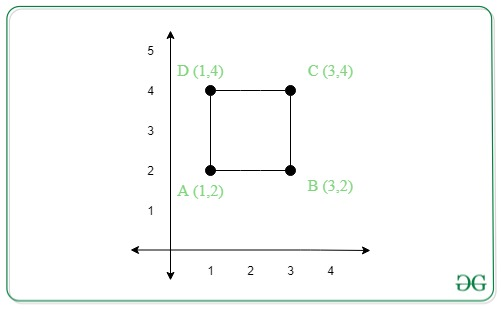GeeksforGeeks App
Open AppBrowser
Continue

# Find the remaining vertices of a square from two given vertices

Given the coordinates of any two vertices of a square (X1, Y1) and (X2, Y2), the task is to find the coordinates of the other two vertices. If a square cannot be formed using these two vertices, print -1.

Examples:

Input: X1 = 1, Y1 = 2, X2 = 3, Y2 = 4
Output: (1, 4), (3, 2)
Explanation:From the above figure the other two vertices of the square will be (1, 4) and (3, 2).

Input: X1 = -5, Y1 = 5, X2 = 5, Y2 = -5
Output: (-5, -5), (5, 5)

Approach: The approach is based on the fact that the length of all the sides of a square are equal. If no such vertices can be obtained for which the length of all the sides become equal, then print “-1”. Follow the steps below to solve the problem:

• The given two vertices can either be the vertices of the side of the square or the vertices of the diagonal.
• If the x-coordinates of the given two vertices are equal then the coordinates of the other two vertices will be:

(X1 + Y2 – Y1, Y1) and (X2 + Y2 – Y1, Y2

• If the y-coordinates of the given two vertices are equal, then the coordinates of the other two vertices will be:

(X1, Y1 + X2 – X1) and (X2, Y2 + X2 – X1)

• Otherwise, the given coordinates are of the diagonal of the square. Therefore, the coordinates of the other two vertices will be:

(X1, Y2) and (X2, Y1)

Below is the implementation of the above approach:

## C++

 `// C++ program for the above approach` `#include ``#include ``using` `namespace` `std;` `// Function to find the remaining``// vertices of a square``void` `findVertices(``int` `x1, ``int` `y1,``                  ``int` `x2, ``int` `y2)``{``    ``// Check if the x-coordinates``    ``// are equal``    ``if` `(x1 == x2) {``        ``cout << (x1 + y2 - y1)``             ``<< ``", "` `<< y1 << endl;` `        ``cout << (x2 + y2 - y1)``             ``<< ``", "` `<< y2;``    ``}` `    ``// Check if the y-coordinates``    ``// are equal``    ``else` `if` `(y1 == y2) {``        ``cout << x1 << ``", "``             ``<< (y1 + x2 - x1)``             ``<< endl;` `        ``cout << x2 << ``", "``             ``<< (y2 + x2 - x1);``    ``}` `    ``// If the given coordinates``    ``// forms a diagonal of the square``    ``else` `if` `(``abs``(x2 - x1)``             ``== ``abs``(y2 - y1)) {` `        ``cout << x1 << ``", "` `<< y2``             ``<< endl;``        ``cout << x2 << ``", "` `<< y1;``    ``}` `    ``// Otherwise``    ``else` `        ``// Square does not exist``        ``cout << ``"-1"``;``}` `// Driver Code``int` `main()``{``    ``// Given two vertices``    ``int` `x1 = 1, y1 = 2;``    ``int` `x2 = 3, y2 = 4;``    ``findVertices(x1, y1, x2, y2);` `    ``return` `0;``}`

## Java

 `// Java program for the above approach``import` `java.util.*;` `class` `GFG{` `// Function to find the remaining``// vertices of a square``static` `void` `findVertices(``int` `x1, ``int` `y1,``                         ``int` `x2, ``int` `y2)``{``    ` `    ``// Check if the x-coordinates``    ``// are equal``    ``if` `(x1 == x2)``    ``{``        ``System.out.print((x1 + y2 - y1) +``                        ``", "` `+ y1 + ``"\n"``);` `        ``System.out.print((x2 + y2 - y1) +``                        ``", "` `+ y2);``    ``}` `    ``// Check if the y-coordinates``    ``// are equal``    ``else` `if` `(y1 == y2)``    ``{``        ``System.out.print(x1 + ``", "` `+``                        ``(y1 + x2 - x1) + ``"\n"``);` `        ``System.out.print(x2 + ``", "` `+``                        ``(y2 + x2 - x1));``    ``}` `    ``// If the given coordinates``    ``// forms a diagonal of the square``    ``else` `if` `(Math.abs(x2 - x1) ==``             ``Math.abs(y2 - y1))``    ``{``        ``System.out.print(x1 + ``", "` `+ y2 + ``"\n"``);``        ``System.out.print(x2 + ``", "` `+ y1);``    ``}` `    ``// Otherwise``    ``else` `        ``// Square does not exist``        ``System.out.print(``"-1"``);``}` `// Driver Code``public` `static` `void` `main(String[] args)``{``    ` `    ``// Given two vertices``    ``int` `x1 = ``1``, y1 = ``2``;``    ``int` `x2 = ``3``, y2 = ``4``;``    ` `    ``findVertices(x1, y1, x2, y2);``}``}` `// This code is contributed by Amit Katiyar`

## Python3

 `# Python3 program for the above approach` `# Function to find the remaining``# vertices of a square``def` `findVertices(x1, y1, x2, y2):``    ` `    ``# Check if the x-coordinates``    ``# are equal``    ``if` `(x1 ``=``=` `x2):``        ``print``((x1 ``+` `y2 ``-` `y1), ``","``, y1)` `        ``print``((x2 ``+` `y2 ``-` `y1), ``","``, y2)` `    ``# Check if the y-coordinates``    ``# are equal``    ``elif` `(y1 ``=``=` `y2):``        ``print``(x1, ``","``, (y1 ``+` `x2 ``-` `x1))` `        ``print``(x2, ``","``, (y2 ``+` `x2 ``-` `x1))` `    ``# If the given coordinates``    ``# forms a diagonal of the square``    ``elif` `(``abs``(x2 ``-` `x1) ``=``=` `abs``(y2 ``-` `y1)):``        ``print``(x1, ``","``, y2)``        ``print``(x2, ``","``, y1)` `    ``# Otherwise``    ``else``:` `        ``# Square does not exist``        ``print``(``"-1"``)` `# Driver Code``if` `__name__ ``=``=` `'__main__'``:``    ` `    ``# Given two vertices``    ``x1 ``=` `1``    ``y1 ``=` `2``    ``x2 ``=` `3``    ``y2 ``=` `4``    ` `    ``findVertices(x1, y1, x2, y2)` `# This code is contributed by mohit kumar 29`

## C#

 `// C# program for the above approach``using` `System;``class` `GFG{` `// Function to find the remaining``// vertices of a square``static` `void` `findVertices(``int` `x1, ``int` `y1,``                         ``int` `x2, ``int` `y2)``{   ``  ``// Check if the x-coordinates``  ``// are equal``  ``if` `(x1 == x2)``  ``{``    ``Console.Write((x1 + y2 - y1) +``                  ``", "` `+ y1 + ``"\n"``);` `    ``Console.Write((x2 + y2 - y1) +``                  ``", "` `+ y2);``  ``}` `  ``// Check if the y-coordinates``  ``// are equal``  ``else` `if` `(y1 == y2)``  ``{``    ``Console.Write(x1 + ``", "` `+``                  ``(y1 + x2 - x1) + ``"\n"``);` `    ``Console.Write(x2 + ``", "` `+``                  ``(y2 + x2 - x1));``  ``}` `  ``// If the given coordinates``  ``// forms a diagonal of the square``  ``else` `if` `(Math.Abs(x2 - x1) ==``           ``Math.Abs(y2 - y1))``  ``{``    ``Console.Write(x1 + ``", "` `+ y2 + ``"\n"``);``    ``Console.Write(x2 + ``", "` `+ y1);``  ``}` `  ``// Otherwise``  ``else` `    ``// Square does not exist``    ``Console.Write(``"-1"``);``}` `// Driver Code``public` `static` `void` `Main(String[] args)``{``  ``// Given two vertices``  ``int` `x1 = 1, y1 = 2;``  ``int` `x2 = 3, y2 = 4;``  ``findVertices(x1, y1, x2, y2);``}``}` `// This code is contributed by Rajput-Ji`

## Javascript

 ``

Output

```1, 4
3, 2```

Time Complexity: O(1)
Auxiliary Space: O(1)

My Personal Notes arrow_drop_up